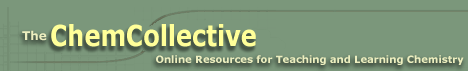# Electrochemistry

-Introduction

Step 1:

-Investigating redox reactions

-Practice with redox reactions

-Reduction tendencies of metal ions

Step 2:

-Electron transfer

-Electrochemical cell

-Practice with cells

-Powering a stopwatch

Step 3:

-Measuring potentials

-Calculating potentials

-Practice with potentials

-Applying potentials

Step 4:

-Non-standard conditions

-Practice with non-standard cells

Feedback Form:

Electrochemistry Tutorial: Galvanic Cells and the Nernst Equation >> Step 1: Investigating redox reactions

 < Previous | Next >

# Electrochemistry: Galvanic Cells and the Nernst Equation

## Step 1: - Investigating redox reactions of some metals and solutions of metal salts

Electrochemistry is often defined as the study of interchange of chemical and electrical energy. In this step we will investigate one aspect of this process: the generation of an electrical current from a spontaneous oxidation-reduction (redox) reaction.

In this step, we will investigate redox reactions between metals and salt solutions. The following movie shows an experiment that tells us whether there is a redox reaction between a metal (Zn) and a metal ion (Cu2+).

The following activity shows the results of a number of experiments similar to that carried out in the above movie. From the results of these experiments, you will:

1. Determine if a spontaneous reaction occurred.
2. If a spontaneous reaction does occur, use the drop down menus to create a balanced redox equation corresponding to this reaction.
3. Finally you will write the half-reactions corresponding to this overall reaction. Half-reactions break the reaction into the oxidation and reduction portions. For instance, the reaction between Zn and Cu2+ corresponds to the following half-reactions:

 Zn(s) --> Zn2+(aq) + 2 e- Oxidation Cu2+(aq) + 2 e- --> Cu(s) Reduction Zn(s) + Cu2+(aq) --> Zn2+(aq) + Cu(s) Full redox reaction

This breaking of a redox reaction into half-reactions is one of the most powerful ideas in redox chemistry, and is the basis for much of what we will be doing in the remainder of this activity.

Activity: Investigating redox reactions.

Note: This activity requires the Flash Player. To ensure the simulation runs properly, please make sure that you have the flash player installed.

 < Previous | Next >